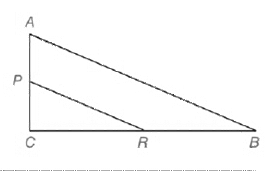# In Exercises 16 and 17, complete the statements and reasons in each proof. Given: △ A B C , P is the midpoint of A C - , and R is the midpoint of C B - . Prove: ∠ P R C ≅ ∠ B Statements Reasons 1.△ ABC 2. ∠ C ≅ ∠ C 3. P is the midpoint of A C - , and R is the midpoint of C B - 4. P C A C = 1 2 and C R C B = 1 2 5. P c A C = C R C B 6. △ C P R ~ △ C A B 7.______________________ 1.________________________ 2. ________________________ 3. ________________________ 4. Definition of midpoint 5._________________________ 6._________________________ 7. CASTC### Elementary Geometry For College St...

7th Edition
Alexander + 2 others
Publisher: Cengage,
ISBN: 9781337614085### Elementary Geometry For College St...

7th Edition
Alexander + 2 others
Publisher: Cengage,
ISBN: 9781337614085

#### Solutions

Chapter
Section
Chapter 5.CT, Problem 17CT
Textbook Problem

## In Exercises 16 and 17, complete the statements and reasons in each proof.Given: △ A B C ,   P is the midpoint of A C - , and R is the midpoint of C B - .Prove: ∠ P R C ≅ ∠ BStatements Reasons 1.△ABC2. ∠ C ≅ ∠ C 3.P is the midpoint of A C - , and R is the midpoint of C B - 4. P C A C = 1 2 and C R C B = 1 2 5. P c A C = C R C B 6. △ C P R ~ △ C A B 7.______________________ 1.________________________2. ________________________3. ________________________4. Definition of midpoint5._________________________6._________________________7. CASTC

Expert Solution
To determine

To complete:

The missing statements and reasons.

### Explanation of Solution

Given: ABC,

P is the midpoint of AC¯, and R is the midpoint of CB¯.

To prove: PRCB

Given figure,

 Statements Reasons 1. △ABC2. ∠C≅∠C3. P is the midpoint of AC¯, and R is the midpoint of CB¯4. PCAC=12 and CRCB=125. PCAC=CRCB6. △CPR~△CAB7. ______________________ 1. ________________________2. ________________________3. ________________________4. Definition of midpoint5. _________________________6. _________________________7. CASTC

Proof:

Consider the triangles CAB and CPR,

Given, P is the midpoint of AC¯, and R is the midpoint of CB¯

### Want to see the full answer?

Check out a sample textbook solution.See solution

### Want to see this answer and more?

Bartleby provides explanations to thousands of textbook problems written by our experts, many with advanced degrees!

See solution